# Comparing Fractions

Here we will learn about comparing fractions, including how to compare fractions with different denominators to work out which of two fractions is the smaller fraction and which is the larger fraction.

There are also comparing fractions worksheets based on Edexcel, AQA and OCR exam questions, along with further guidance on where to go next if you’re still stuck.

## What is comparing fractions?

Comparing fractions allows us to compare the size of a fraction with other fractions.

To do this we can use equivalent fractions. We can also use inequality symbols < (less than) and > (greater than) to help us write about the comparison.

For example, which fraction is larger, \frac{2}{5} or \frac{3}{8}?

In the diagrams, the fractions are indicated by the white sections.

Using equivalent fractions we can convert both fractions so they have a common denominator. The least common denominator (lcd), or, the lowest common multiple (least common multiple) of the denominators.

The lowest common multiple of 5 and 8 is 40.

\frac{2}{5}=\frac{2\times 8}{5\times 8}=\frac{16}{40}

and

\frac{3}{8}=\frac{3\times 5}{8\times 5}=\frac{15}{40}

\frac{16}{40} has a larger numerator than \frac{15}{40}.

Therefore \frac{2}{5} is the larger number.

We can write this as

\frac{2}{5}>\frac{3}{8}.

### What is comparing fractions?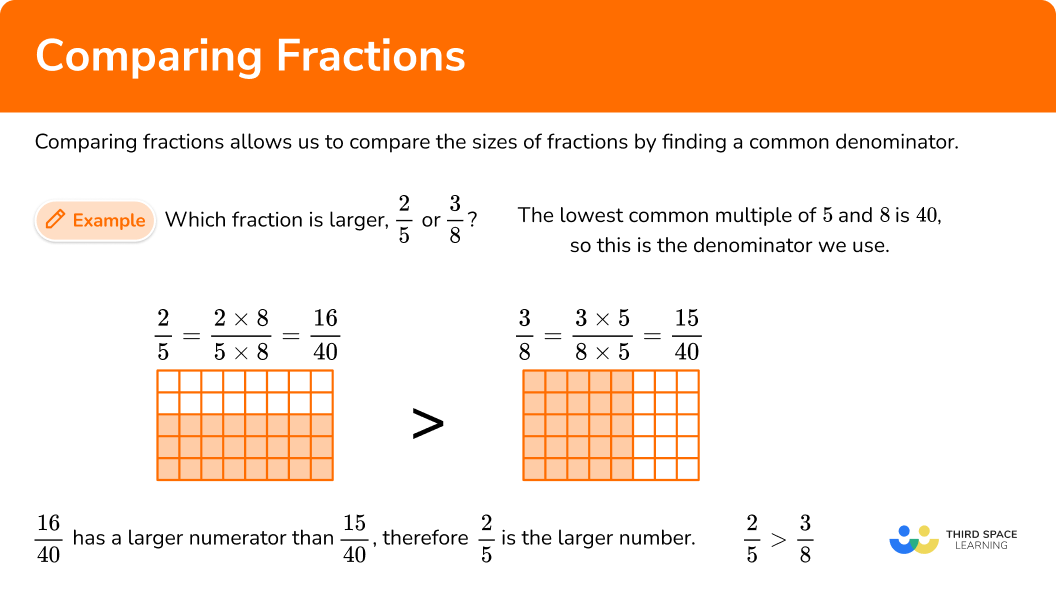## How to compare fractions

In order to compare fractions:

1. See if the fractions have the same denominators.
2. Make equivalent fractions if needed.
3. Write the answer using the original fractions.

### Explain how to compare fractions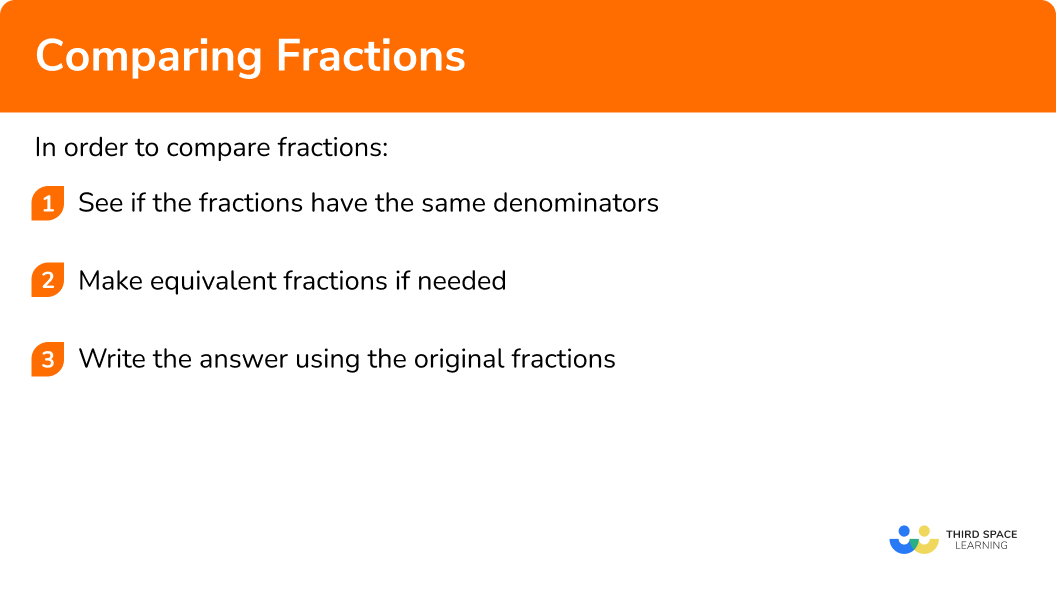### Related lessons on fractions

Comparing fractions is part of our series of lessons to support revision on fractions. You may find it helpful to start with the main fractions lesson for a summary of what to expect, or use the step by step guides below for further detail on individual topics. Other lessons in this series include:

## Comparing fractions examples

### Example 1: like numerators

Which is the smallest fraction \frac{3}{8} or \frac{3}{10}?

1. See if the fractions have the same denominators.

The fractions do not have the same denominators (bottom numbers), but they have the same numerators (top numbers).

We can use the fact that eighths are bigger than tenths.

So the \frac{3}{8} will be larger than \frac{3}{10}.

2Make equivalent fractions if needed.

We do not need to use equivalent fractions. The fraction with the smaller denominator will be the larger number.

3Write the answer using the original fractions.

\frac{3}{10} is the smaller number.

We can write this as

\frac{3}{8}>\frac{3}{10}.

### Example 2: like denominators and unlike numerators

Which is the smallest fraction \frac{2}{7} or \frac{5}{7}?

See if the fractions have the same denominators.

Make equivalent fractions if needed.

Write the answer using the original fractions.

### Example 3: unlike denominators

Which is the smallest fraction \frac{2}{5} or \frac{3}{4}?

See if the fractions have the same denominators.

Make equivalent fractions if needed.

Write the answer using the original fractions.

### Example 4: different denominators

Which is the largest fraction \frac{4}{9} or \frac{5}{11}?

See if the fractions have the same denominators.

Make equivalent fractions if needed.

Write the answer using the original fractions.

### Example 5: different denominators – improper fractions

Which is the largest value \frac{14}{3} or \frac{7}{2}?

See if the fractions have the same denominators.

Make equivalent fractions if needed.

Write the answer using the original fractions.

### Example 6: different denominators – mixed numbers

Which is the largest value 2\frac{1}{3} or 2\frac{2}{7}?

See if the fractions have the same denominators.

Make equivalent fractions if needed.

Write the answer using the original fractions.

### Common misconceptions

• Use inequality symbols correctly

You may be asked to use inequality symbols. Make sure you get them the right way around, < is “less than” and > is “greater than”.

• Equivalent fractions is only one way to compare fractions

You do not have to use equivalent fractions to compare fractions. It may be easier to use decimals instead. For example, comparing \frac{1}{2} and \frac{3}{10}. These fractions as decimals would be 0.5 and 0.3. Therefore \frac{1}{2} is the smallest fraction and \frac{3}{10} is the larger fraction.

### Practice comparing fractions questions

1. Which is the smallest value?

\frac{3}{5} \hspace{1cm} \frac{3}{17} \hspace{1cm} \frac{3}{4} \hspace{1cm} \frac{3}{11}

\frac{3}{5}\frac{3}{4}\frac{3}{17}\frac{3}{11}All the fractions have the same numerator. We can then compare the denominators. 17 is the largest denominator, so \frac{3}{17} is the smallest.

2. Which is the largest fraction?

\frac{8}{15} \hspace{1cm} \frac{11}{15} \hspace{1cm} \frac{7}{15} \hspace{1cm} \frac{13}{15}

\frac{11}{15}\frac{13}{15}\frac{8}{15}\frac{7}{15}All the fractions have the same denominators. We can then compare the numerators. 13 is the largest numerator, so \frac{13}{15} is the largest.

3. Which is the largest fraction?

\frac{4}{5} \hspace{1cm} \frac{1}{2} \hspace{1cm} \frac{3}{4} \hspace{1cm} \frac{7}{10}

\frac{7}{10}\frac{4}{5}\frac{1}{2}\frac{3}{4}All the fractions have different denominators. We can use equivalent fractions so that all the fractions have the same denominators and we can compare the fractions more easily.

\frac{4}{5}=\frac{16}{20}

\frac{1}{2}=\frac{10}{20}

\frac{3}{4}=\frac{15}{20}

\frac{7}{10}=\frac{14}{20}

We can then compare the numerators. 16 is the largest numerator, so \frac{4}{5} is the largest.

4. Which is the smallest fraction?

\frac{2}{3} \hspace{1cm} \frac{5}{6} \hspace{1cm} \frac{3}{4} \hspace{1cm} \frac{11}{12}

\frac{5}{6}\frac{11}{12}\frac{3}{4}\frac{2}{3}All the fractions have different denominators. We can use equivalent fractions so that all the fractions have the same denominators and we can compare the fractions more easily.

\frac{2}{3}=\frac{8}{12}

\frac{5}{6}=\frac{10}{12}

\frac{3}{4}=\frac{9}{12}

\frac{11}{12}

We can then compare the numerators. 8 is the smallest numerator, so \frac{2}{3} is the smallest fraction.

5. Which is the smallest number?

\frac{10}{3} \hspace{1cm} \frac{17}{7} \hspace{1cm} \frac{9}{2} \hspace{1cm} \frac{11}{4}

\frac{9}{2}\frac{11}{4}\frac{17}{7}\frac{10}{3}We can rewrite these improper fractions as mixed numbers first.

\frac{10}{3}=3\frac{1}{3}

\frac{17}{7}=2\frac{3}{7}

\frac{9}{2}=4\frac{1}{2}

\frac{11}{4}=2\frac{3}{4}

We can see that we need to further look at the numbers with 2 as the whole number part. We could write them using equivalent fractions, but \frac{3}{4} is greater than a half and \frac{3}{7} is less than a half. So, \frac{17}{7} will be the smallest number.

6. Which is the largest number?

7\frac{1}{2} \hspace{1cm} 7\frac{7}{10} \hspace{1cm} 7\frac{13}{20} \hspace{1cm} 7\frac{3}{5}

7\frac{1}{2}7\frac{3}{5}7\frac{13}{20}7\frac{7}{10}We can rewrite these improper fractions so that the fraction parts have a common denominator of 20.

7\frac{1}{2}=7\frac{10}{20}

7\frac{7}{10}=7\frac{14}{20}

7\frac{13}{20}

7\frac{3}{5}=7\frac{12}{20}

The largest number will be 7\frac{7}{10}.

### Comparing fractions GCSE questions

1. Compare these unit fractions.

\frac{1}{6} \hspace{1cm} \frac{1}{3} \hspace{1cm} \frac{1}{9} \hspace{1cm} \frac{1}{4}

(a) Write down the smallest value.

(b) Write down the largest value.

(2 marks)

(a) \frac{1}{9}

(1)

(b) \frac{1}{3}

(1)

2. Complete these statements using the correct symbol

<, \ > or =.

(a)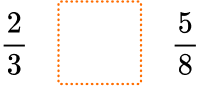(b)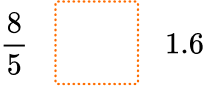(c)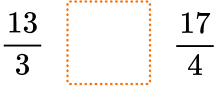(3 marks)

(a) >

(1)

(b) =

(1)

(c) >

(1)

3. Compare these numbers.

3\frac{5}{8} \hspace{1cm} \frac{7}{2} \hspace{1cm} 3.4 \hspace{1cm} \frac{25}{7}

(a) Write down the smallest value.

(b) Write down the largest value.

(2 marks)

(a) 3.4

(1)

(b) 3\frac{5}{8}

(1)

4. Robbie says that “two-thirds is smaller than seven-tenths”.

(2 marks)

For using equivalent fractions.

For example, \frac{2}{3} = \frac{20}{30} \ and \ \frac{7}{10} = \frac{21}{30}.

(1)

Yes Robbie is correct, with correct workings.

(1)

## Learning checklist

You have now learned how to:

• Compare fractions with the same numerators
• Compare fractions with the same denominators
• Compare fractions with different denominators

## Still stuck?

Prepare your KS4 students for maths GCSEs success with Third Space Learning. Weekly online one to one GCSE maths revision lessons delivered by expert maths tutors.

Find out more about our GCSE maths tuition programme.